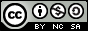- 目录 -
LeetCode 546 移除盒子(区间dp，记忆化搜索)

输入：boxes = [1,3,2,2,2,3,4,3,1]

[1, 3, 2, 2, 2, 3, 4, 3, 1]
----> [1, 3, 3, 4, 3, 1] (3*3=9 分)
----> [1, 3, 3, 3, 1] (1*1=1 分)
----> [1, 1] (3*3=9 分)
----> [] (2*2=4 分)


• 1 <= boxes.length <= 100
• 1 <= boxes[i] <= 100

## 思路

• $dp[l][r][k]=dp[l][r-1]+(k+1)^2$

• $dp[l][r][k]=max(dp[l][r][k],dp[l][i][k+1]+dp[i+1][r-1])$

## 代码

  1 2 3 4 5 6 7 8 9 10 11 12 13 14 15 16 17 18 19 20 21 22 23 24  class Solution { public: int dp; int removeBoxes(vector &boxes) { memset(dp, 0, sizeof(dp)); return dfs(boxes, 0, boxes.size() - 1, 0); } int dfs(vector &nums, int l, int r, int k) { if (l > r) return 0; if (dp[l][r][k]) return dp[l][r][k]; while (r > 0 && nums[r] == nums[r - 1]) r--, k++; dp[l][r][k] = dfs(nums, l, r - 1, 0) + (k + 1) * (k + 1); for (int i = l; i < r; i++) if (nums[i] == nums[r]) dp[l][r][k] = max(dp[l][r][k], dfs(nums, l, i, k + 1) + dfs(nums, i + 1, r - 1, 0)); return dp[l][r][k]; } };